## Abstract

Parallel transmission lines are difficult to protect due to mutual coupling between circuits. This paper proposes a Naïve Bayes classifier (NBC) based fault detection and classification technique for protection of parallel transmission line involving inter-circuit faults. NBC is a good classification tool for larger data sets as the training process takes less time with greater accuracy. Input given to the fault detection module is the fundamental components of three phase current signals of both circuits. Input given to the fault phase identification and fault classification module is the fundamental component of three phase current signals and zero sequence currents of both the circuits. Seven separate classifiers are designed for fault phase identification for A1, B1, C1, A2, B2, C2 and G. From fault phase identification module faults are classified. Accuracy of the proposed method is 100% for fault detection and 99.99% for classification of fault from all the tested fault cases. Response time of the proposed method is within 10 ms for all the fault cases studied.

## Keywords

Fundamental components of current; Naïve Bayes classifier; Fault detection; Fault phase identification; Fault classification

## 1. Introduction

Protection of transmission lines is necessary to provide efficient and reliable power flow. The most important protection tasks of transmission lines include fault detection and classification. Fault detection in transmission lines is done by many researchers using different methods. Recently, the wavelet transform has been successfully applied in many fields. High impedance faults (HIFs) detection in high-voltage transmission lines use wavelet transform in . HIFs are also detected using decision trees using current magnitudes of the second, third, and fifth harmonics, and the phase of the third harmonics in . The technique is based on using the absolute sum value of coefficients in multi-resolution signal decomposition based on the discrete wavelet transform. A cumulative-sum-based fault detection algorithm is also proposed for the power system relaying application in . A technique based on an embedding theorem is proposed which shows that a time series can be mapped to a higher dimensional space called phase space through embedding in . The original signal is decoupled into two parts: normal and disturbance parts. The fault detection is easily achieved as the disturbance part of the signal produces an irregular shape compared to the shape produced from the normal part of the signal.

All the above described methods only detect the fault but did not classify the faults. Fault classification in transmission lines is done using many methods. Fault phase selection and fault classifications are carried out using wavelet transform in  and . Discrete wavelet transform integrated with a fuzzy logic system is designed for fault classification in transmission line in . ANN is also used for fault classification in transmission lines by researchers in . Wavelet combined with ANN is used for fault classification and fault phase selection in  and . Support vector machines are used for classification of faults in transmission lines taking samples of one cycle duration of three line currents and zero sequence current as input to the classifier in . Decision trees are used for classification of fault in single circuit transmission lines. After detecting the exact time of fault inception, the fault type is recognized by means of a decision-tree algorithm which is formerly trained by applying the odd harmonics of the measured signals, up to the 19th . Apart from normal shunt faults inter-circuit faults are also classified by researchers in .

So a method is proposed using Naïve Bayes classifier for detection and classification of fault in parallel transmission lines by taking fundamental components and zero sequence components as input to the classifier. The paper is arranged as follows – Section 2 describes the Naïve Bayes classifier, Section 3 describes how the signals are processed, Section 4 describes the proposed method in detail, Section 5 shows the results and performance of the relay and Section 6 contains the conclusion of the proposed method.

## 2. Naïve Bayes classifier

Bayesian classifiers are statistical classifiers which use supervised learning method to predict class membership probabilities. Bayesian classification is based on Bayes’ theorem which provides practical learning algorithms in which prior knowledge and observed data can be combined. Learning method used in Bayesian classification is probabilistic learning method . It is applied to decision making and inferential statistics that deals with probability inference. The Bayes Theorem says that

 $p(h\vert d)={\frac {P(d\vert h)P(h)}{P(d)}}$
(1)

where

• P(h): prior probability of hypothesis h (Prior belief),
• P(d): prior probability of training data d (Data Evidence),
• p(h/d): probability of h given d (Posterior probability),
• P(d/h): probability of d given h (Likelihood).

Naïve Bayes classification is based on the Bayesian theorem used when the dimensionality of the inputs is high. Naive Bayes classifier uses method of maximum likelihood for classifying the data. Naïve Bayesian classifiers assume that the effect of an attribute value on a given class is independent of the values of the other attributes. This assumption is called class conditional independence .

For a set of variables X: (x1, x2, x3, … , xn), there will be different classes C: C1, C2, C3,  … , Cm where m and n are integers. Naïve Bayes classifier predicts X belongs to Class Ci if and only if

 $P(Ci/X)>P(Cj/X)$
(2)

where i ≠ j   and ${\textstyle P(Ci/X)=P(X/Ci)P(Ci)/P(X)}$.

The class Ci for which P(Ci/X) is maximized is called the maximum posteriori hypothesis. Naïve Bayes classifier is used in this paper as classifier for detection and classification. It consists of two phases training and testing. Training of the network is carried out using different fault cases. After training the network is tested for different fault cases other than that are used in training.

## 3. Signal processing

The single line diagram of the power system network under consideration is shown in Fig. 1. It consists of 400 kV, 50 Hz double circuit transmission line of length 100 km. Three phase source of 400 kV, 50 Hz is connected to bus-1 with 100 kW and 100 kVar load, the short circuit capacity of source is 1250 MVA and X/R ratio is 10. At bus-2 a load of 250 kW and another 3 phase source of 400 kV, 50 Hz, 1250 MVA SCC, and 10 X/R ratio are connected. Three phase currents of both circuits are obtained from the relaying point sampled at a sampling frequency of 1 kHz. From three phase components of current fundamental components and zero sequence currents are extracted. By employing the DFT block and sequence analyzer block the fundamental components of three phase currents and zero sequence currents signals of ckt-1 & 2 are obtained. Three phase currents, its fundamental components and zero sequence currents during A1G fault are shown in Fig. 2. Fig. 2(a) and (b) shows the three phase currents during A1G fault in circuit 1 and circuit 2 respectively. Fig. 2(c) and (d) shows fundamental components and zero sequence components of circuit 1 and circuit 2 respectively.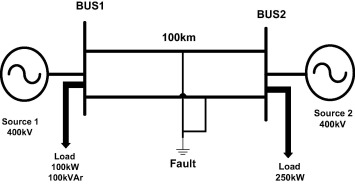Figure 1. Considered double circuit transmission line.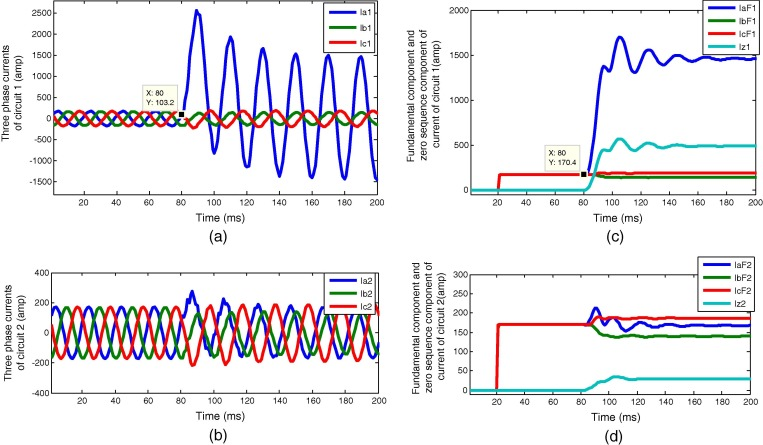Figure 2. (a) Three phase currents of circuit 1. (b) Three phase currents of circuit 2. (c) Fundamental currents and zero sequence currents of circuit 1. (d) Fundamental currents and zero sequence currents of circuit 2.

## 4. Proposed method

Proposed NBC based fault detection and classification method is described in flow diagram 3. Proposed method consists of two modules one for fault detection and other for fault classification. A NBC network is designed using various fault cases in training. Various fault cases used in training and testing are given in Table 1.

Table 1. Variation of different parameters for training and testing.
Parameters Training Testing
Fault locations (L in km) 10 locations in step of 10 km (5–95 km) Different locations (different values between 1 and 99 km)
Fault inception angles (ϕ in °) 0 0, 45, 90, 135, 180, 225, 270, 315 and 360
Fault resistances (Rf in Ω) 0, 10 and 100 0, 5, 10, 50 and 100
Fault type LG, LLG, LL, LLL and inter-circuit faults LG, LLG, LL, LLL and inter-circuit faults

### 4.1. Fault detection module

For fault detection three phase currents of both circuits are obtained from relaying point sampled at 1 kHz. Fundamental components of currents are then extracted from three phase currents. Fault detection module takes fundamental components of current signals of both the circuits as input. For training the network fault cases are taken for different parameters. The network is then trained using NBC with training accuracy 99.98%. Trained NBC network is used to test various fault cases. Output of fault detection module is ‘0’ when no fault and ‘1’ when there is a fault in the system.

### 4.2. Fault classification module

Fault detection module detects whether there is a fault in the network or not. If there is a fault in the system then the fault phases are identified and faults are classified as shown in Fig. 3. Fault classification module is designed for each phase A1, B1, C1, A2, B2, C2 and G for ground. For each phase a NBC network is designed. Fault classification module takes fundamental components and zero sequence components of current signals of both the circuit as input. For training the network fault cases are taken for different parameters. The network is then trained using NBC with training accuracy 99.97%. Trained NBC network is used to test various fault cases. Output of each phase is ‘0’ when no fault and ‘1’ when there is a fault in any of the phases. After the fault phases are identified, fault classification occurs.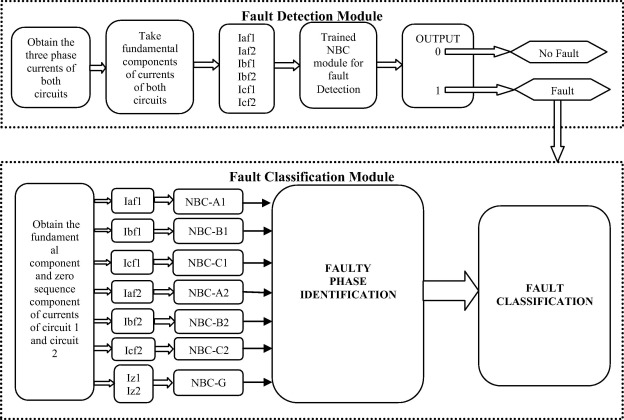Figure 3. Flow diagram of the proposed method.

Various fault cases are tested to check the performance of the proposed relay. Different fault cases used for testing purpose varying different parameters are shown in Table 1. Performance of the proposed NBC based fault detector and classifier is discussed in next section.

## 5. Results and discussions

Proposed NBC based fault detection and classification technique is tested for various fault cases. Total number of fault cases used for testing the proposed NBC based method is 2200 cases. Variation of parameters considered is fault type, fault location, fault inception angle, inter-circuit faults and fault resistance, etc.

### 5.1. Different fault type

Proposed method is tested for different fault types in both the circuits. Fig. 4 shows the inputs and outputs of fault detection and classification during A2G fault at 77 km with Rf = 0.001, Фi = 0° at 80 ms. Fig. 4(a) and (b) shows the fundamental components and zero sequence components of current signals for circuit 1 and circuit 2 respectively. As the fault is A2G fault fundamental component of A2 phase current is higher than the other phases. Also the zero sequence current of circuit 2 is higher than circuit 1. Fig. 4(c) shows the output of fault detection which is ‘0’ up to 80 ms of time. When fault occurs at 80 ms it becomes high ‘1’ at 86 ms of time. So Proposed relay takes 6 ms (86 − 80 = 6) time for detection of fault. So response time for detection of fault is considered as 6 ms. Fig. 4(d) shows the classification output for the A2G fault. Phases A2 and G become ‘1’ after occurrence of fault at 80 ms time shows that there is A2G fault. Response times for detection of fault for various faults are shown in Table 2. Fault detection time for the proposed method is within 10 ms (half cycle) for all the fault cases tested. Table 3 shows the percentage accuracy of fault detection and classification for different types of fault. Fault detection accuracy is 100% for all types of fault. Fault classification accuracy is 100% for all except LG fault which is 99.99%.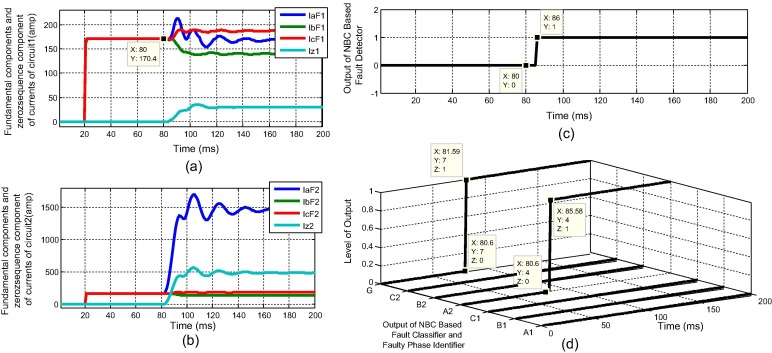Figure 4. (a) Fundamental and zero sequence currents of circuit 1. (b) Fundamental and zero sequence currents of circuit 2. (c) Output of fault detection. (d) Output of fault classification for A2G faults at 77 km with Rf = 0.001, Фi = 0° at 80 ms.

Table 2. Response time case of different fault types.
Fault type circuit 1 Response time (ms) Fault type circuit 2 Response time (ms)
A1G 6 A2G 6
B1G 5 B2G 5
C1G 10 C2G 10
A1B1G 4 A2B2G 4
B1C1G 5 B2C2G 5
C1A1G 6 C2A2G 6
A1B1 4 A2B2 4
B1C1 5 B2C2 5
C1A1 8 C2A2 8
A1B1C1 5 A2B2C2 4

Table 3. Performance in case of different fault types.
Fault type Number of fault cases Fault detection accuracy (%) Fault classification accuracy (%)
LG 600 100 99.90
LLG 600 100 100
LL 600 100 100
LLL 200 100 100

### 5.2. Varying fault location

Proposed NBC method is tested for various fault locations of line. Test results of variation of fault locations are shown in Table 4. Locations are represented in terms of line length L for different fault cases. Accuracy of fault detection is 100% for all the test cases. Accuracy of fault classification is 100% except some near end and far end faults which are 99.99%. Fault detection time is within 10 ms (half cycle) for all the fault cases.

Table 4. Performance in case of different fault locations.
Fault location in L Number of fault cases Fault detection accuracy (%) Fault classification accuracy (%) Response time (ms)
0–0.1L 230 100 99.99 ⩽10
0.1–0.2L 300 100 100 ⩽10
0.2–0.3L 110 100 100 ⩽10
0.3–0.4L 140 100 100 ⩽10
0.4–0.5L 190 100 100 ⩽10
0.5–0.6L 170 100 100 ⩽10
0.6–0.7L 180 100 100 ⩽10
0.7–0.8L 230 100 100 ⩽10
0.8–0.9L 300 100 100 ⩽10
0.9–1L 350 100 99.99 ⩽10

### 5.3. Varying fault inception angle

NBC based fault detection and classification method is tested for various fault inception angles. Test results of variation of fault inception angles are shown in Table 5. Accuracy of fault detection is 100% for all the test cases. Accuracy of fault classification is 100% except some fault cases which are 99.99%. Fault detection time is within 10 ms (half cycle) for all the fault cases. Fig. 5 shows the fault detection and classification output for A1B1 faults at 91 km with Rf = 0.001, Фi = 180° at 90 ms. Fig. 5(a) shows the outputs of fault detection which is ‘0’ up to 90 ms show there is no fault. When fault occurs at 90 ms output starts increasing and reaches to ‘1’ at 94 ms and shows there is a fault in system. Fig. 5(b) shows the output of fault classification. Phase A1 and phase B1 changes from ‘0’ to ‘1’ show that there is A1B1 fault.

Table 5. Performance in case of different fault inception angles.
Fault Inception Angle (°) Number of fault cases Fault detection accuracy (%) Fault classification accuracy (%) Response time (ms)
0 1000 100 100 ⩽10
45 200 100 99.99 ⩽10
90 300 100 100 ⩽10
135 150 100 99.99 ⩽10
180 150 100 100 ⩽10
225 150 100 100 ⩽10
270 100 100 100 ⩽10
315 50 100 100 ⩽10
360 100 100 100 ⩽10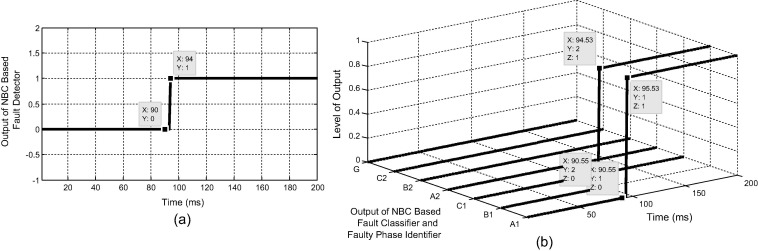Figure 5. (a) Output of fault detection. (b) Output of fault classification for A1B1 faults at 91 km with Rf = 0.001, Фi = 180° at 90 ms.

### 5.4. Varying fault resistance

The traditional relaying scheme like Mho relay is not able to detect the high impedance faults and the reach of the relay is greatly reduced (under-reaches). So the proposed method is tested by different fault cases with varying fault resistances and one result is compared with Mho relay as shown in Fig. 6. Fig. 6 shows the results of fault detection during A1G fault at 50 km with fault resistance of 100 Ω. Fig. 6(a) shows the output of Mho relay which is not able to detect the high impedance faults, as its impedance trajectory does not enter the protected zone characteristics, while Fig. 6(b) shows the output of fault detection of proposed scheme which detects the fault correctly. Test results of variation of fault resistance of proposed method are shown in Table 6. Accuracy of fault detection is 100% for all the test cases. Accuracy of fault classification is 100% except some faults which are 99.99%. Fault detection time is within 10 ms (half cycle) for all the fault cases.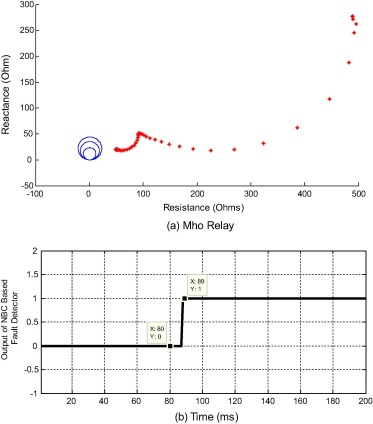Figure 6. Output of fault detection (a) Mho relay and (b) NBC based scheme during A1G faults at 50 km with Rf = 100, Фi = 0° at 80 ms time.

Table 6. Performance in case of different fault resistances.
Fault resistance (Ω) Number of fault cases Fault detection accuracy (%) Fault classification accuracy (%) Response time (ms)
0 700 100 100 ⩽10
5 200 100 100 ⩽10
10 450 100 100 ⩽10
50 250 100 100 ⩽10
100 600 100 99.90 ⩽10

### 5.5. Inter-circuit faults

In double circuit transmission lines there is a chance that fault may occur between the phases of two circuits. So in proposed method inter-circuits faults tested for fault detection and classification. Fig. 7 show the fault detection and classification output for B1A2G faults at 13 km with Rf = 0.001, Фi = 0° at 80 ms. Fig. 7(a) shows the outputs of fault detection which is ‘0’ up to 80 ms show there is no fault. When fault occurs at 80 ms output starts increasing and reaches to ‘1’ at 84 ms and shows there is a fault in system. Fig. 7(b) shows the output of fault classification. Phase A2, phase B1 and ground G changes from ‘0’ to ‘1’ show that there is B1A2G inter-circuit fault as it involves phases of both the circuits. Test results of different inter-circuit faults are shown in Table 7. Accuracy of fault detection is 100% for all the test cases. Accuracy of fault classification is 100% except some fault cases which are 99.99%. Fault detection time is within 10 ms (half cycle) for all the fault cases.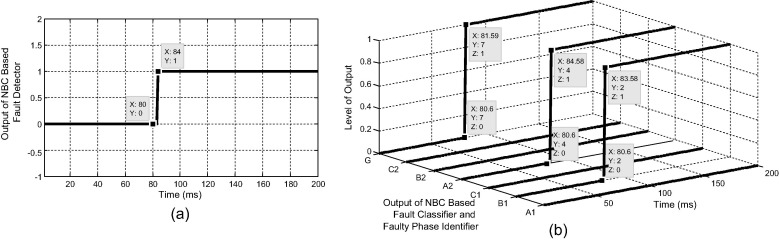Figure 7. (a) Output of fault detection. (b) Output of fault classification for B1A2G faults at 13 km with Rf = 0.001, Фi = 0° at 80 ms.

Table 7. Performance in case of different intercircuit faults.
Fault type Number of fault cases Fault detection accuracy (%) Fault classification accuracy (%) Response time (ms)
A1B2G 40 100 100 ⩽10
A1C2G 30 100 100 ⩽10
B1A2G 30 100 100 ⩽10
B1C2G 40 100 100 ⩽10
C1A2G 30 100 100 ⩽10
C1B2G 30 100 100 ⩽10

Further in order to compare the proposed scheme with conventional distance relaying scheme based on mho relay, another B1A2G fault at 50 km with Rf = 0.001, Фi = 0° at 80 ms is simulated and the three phase current measured at sending end at bus-1 and 2 of the two circuits of double circuit line is depicted in Fig. 8(a). The test result of mho relay with its impedance trajectory is depicted in Fig. 8(b). It can be seen that, the trajectory does not enter the zone-1 but it comes under zone-2. Thus the fault is detected in zone-2; however, the fault was actually at 50 km, which is within the first zone protection boundary. But the mho relay could not detect it in zone-1; thus, it under-reaches. On the other hand, the proposed scheme detects the fault correctly and also identifies the faulted phases A1B2 and ground G accurately as illustrated in Fig. 9(a and b).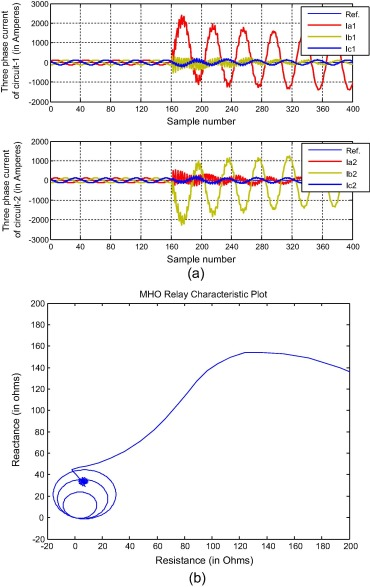Figure 8. During B1A2G earthed intercircuit faults at 50 km with Rf = 0.001, Фi = 0° at 80 ms. (a) Three phase currents of circuit-1 and 2 (b) Impedance trajectory of Mho Relay.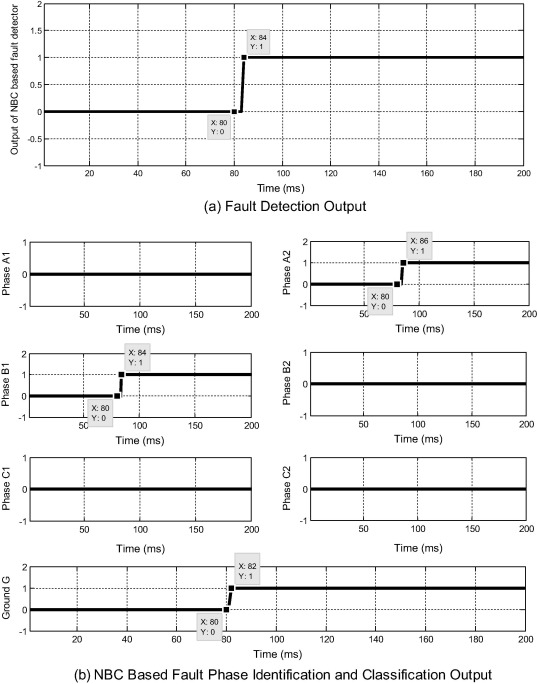Figure 9. During B1A2G earthed inter-circuit faults at 50 km with Rf = 0.001, Фi = 0° at 80 ms output of NBC based scheme (a) fault detection and (b) fault classification.

### 5.6. Far end faults

Proposed method is tested for fault near boundaries to evaluate the reach setting of the relay. Fig. 10 shows the C2A2G fault at 99 km with Rf = 100, Фi = 0° at 80 ms. Fig. 10(a) shows the outputs of fault detection which is ‘0’ up to 80 ms show there is no fault. When fault occurs at 80 ms output starts increasing and reaches to ‘1’ at 89 ms and shows there is a fault in system. Fig. 10(b) shows the output of fault classification. Phase A2, phase C2 and ground G changes from ‘0’ to ‘1’ show that there is A2C2G fault. Table 8 shows the test results of various far end faults. Proposed NBC based method detects and classifies the faults up to 99 km of line. So proposed method can protect 99% of line length and reach setting of the line is 99%.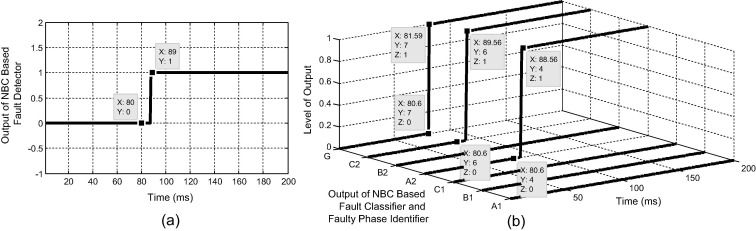Figure 10. (a) Output of fault detection. (b) Output of fault classification for A2C2G faults at 13 km with Rf = 0.001, Фi = 0° at 80 ms.

Table 8. Performance in case of different far end faults.
Fault Location (km) Fault type Fault resistance (Ω) Fault inception angle (°) Response time (ms)
81 A1G 0 0 4
83 A1B1G 5 45 6
85 A1B1 10 90 5
87 A1B1C1 50 135 6.5
89 B2G 100 180 4
91 B2C2G 0 225 7
93 B2C2 5 270 9
95 A2B2C2 10 315 7.5
97 C2G 50 360 4
99 C2A2G 100 0 9

## 6. Conclusion

Proposed Naïve Bayes classifier (NBC) based fault detection and classification technique for protection of double circuit transmission line is not affected by variation of parameters such as fault type, fault location, fault inception angle and fault resistances. Input given to the fault detection, fault phase identification and fault classification module is the fundamental component of three phase current signals and zero sequence currents of both the circuits. One module is designed for fault detection. Seven separate classifiers are designed for fault phase identification for A1, B1, C1, A2, B2, C2 and G. From fault phase identification module faults are classified. Accuracy of the proposed method is 100% for fault detection and 99.99% from all the tested fault cases. Response time is within 10 ms for all the fault cases studied. Advantage of the proposed method is that it detects the fault within half cycle time and accuracy of the proposed method is high.

### Document informationPublished on 12/04/17

Licence: Other

### Document Score0

Views 13
Recommendations 0